# Struggling With Which Statistical Test to Run?

## QI Macros Takes the Guestwork out of Selecting the Right Statistical Test!

### QI Macros Statistical Wizard is Easy to Use

#### 1. Just select your data#### 2. Select the Stat Wizard#### 3. QI Macros does the rest### Decision Trees are Cumbersome

If you are like most people, you have a hard time deciding which statistical test to run. Rather than finding your way through a forest of decision trees, like the ones in Minitab®, QI Macros has built the decision tree right into its code!

Since you select your data first when you use QI Macros, it can choose the most likely statistical analysis tests and run them for you. Even better, QI Macros interprets the results for you too!

#### Use this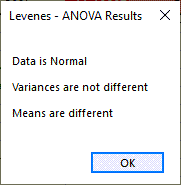#### Not that### How the Statistics Wizard Selects the Right Tests to Run

The Stat Wizard will look at your data to determine if it has 1, 2 or more columns of data. Then, depending on the number of columns and whether the data has decimals or integers, it will run the following statistics:

### 1-Column Example

When you select one column of data, QI Macros Stat Wizard will run Descriptive Statistics and a 1-sample t Test: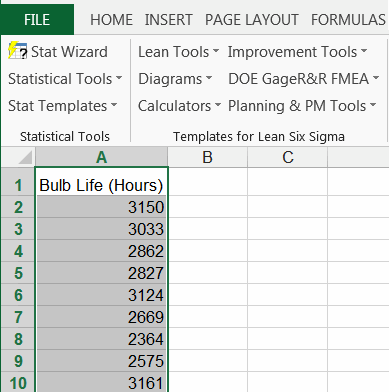Descriptive statistics: note p value is > 0.05 so data is normal.One sample t test: Using Test Mean of 2500 hours you will get: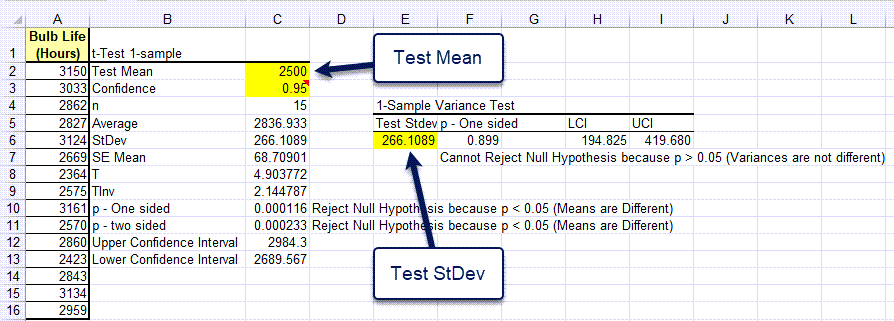### 2-Column Example

By selecting two columns, the Stat Wizard will run an F test, t Test and regression analysis. It may also run an Equivalence test, Chi Square or Fishers Exact test.Stat Wizard runs the F Test to evaluate variances.Since the F test assumes equal variances, the Stat Wizard runs a t test assuming equal variances.Next it runs an Equivalence test for Means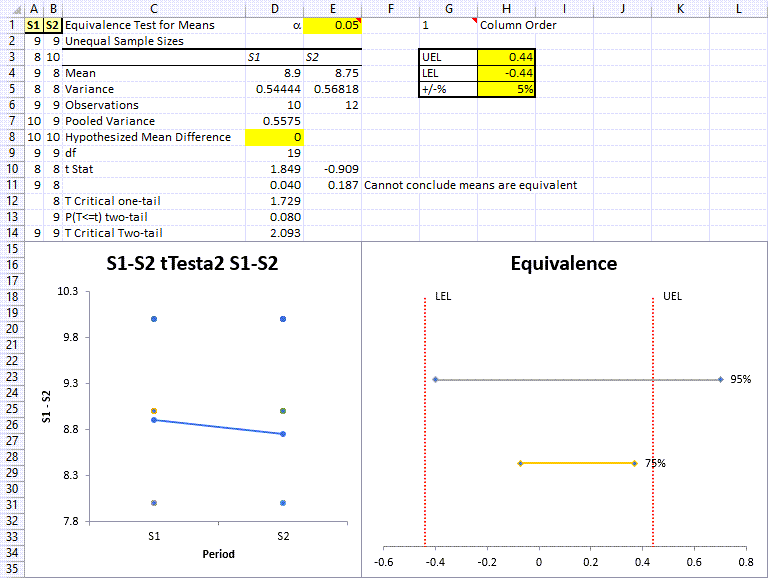Then it runs regression analysis for correlation and fit:Finally, it reports a summary of the results:### 4-Column Example

By selecting the hardwood concentration data below, the Stat Wizard will run a one-way ANOVA to evaluate the means, Levene's test to evaluate the variances and Chi-Squared table to evaluate independence of the variables.

First select your data and then click on QI Macros menu and select Stat Wizard.The Stat Wizard will run ANOVA Single Factor analysis:Then run Descriptive Statistics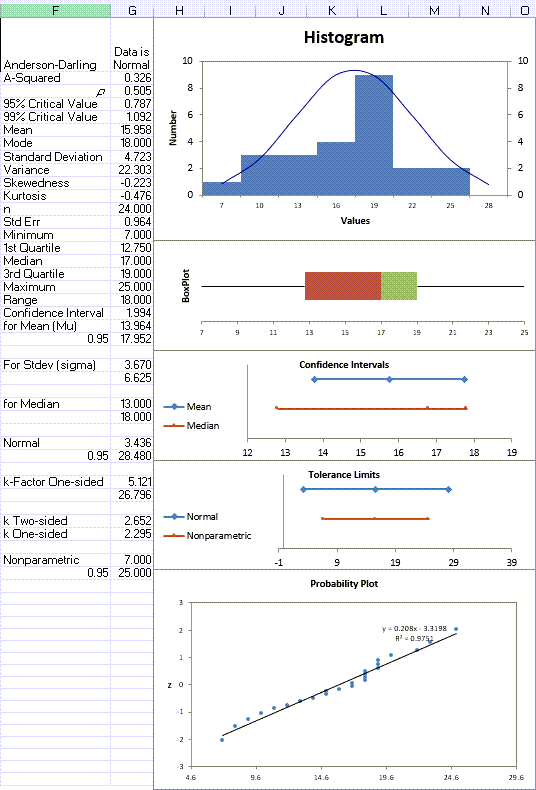Then run Chi-Squared Table to evaluate independence of the variables: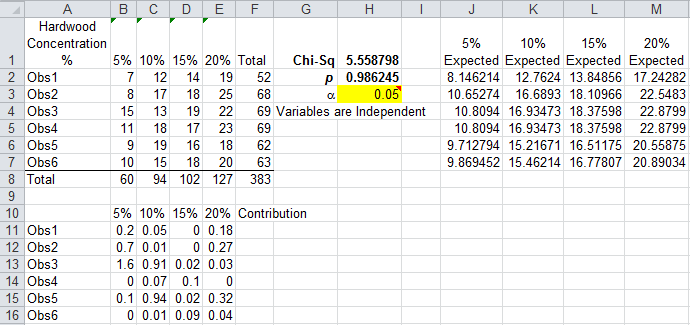Then run Levene's Test to evaluate Variances:Then report a summary of the results: